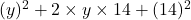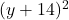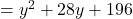## Complete the square. Fill in the number that makes the polynomial a perfect-square quadratic. y2 + 28y +

Question

Complete the square. Fill in the number that makes the polynomial a perfect-square quadratic. y2 + 28y +

in progress 0
2 weeks 2021-09-01T18:05:15+00:00 1 Answers 0 views 0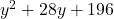Step-by-step explanation:

We are given that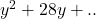We have to fill in the number that makes the polynomial a perfect square quadratic.

We know that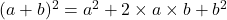Using the formula

Expand the expression